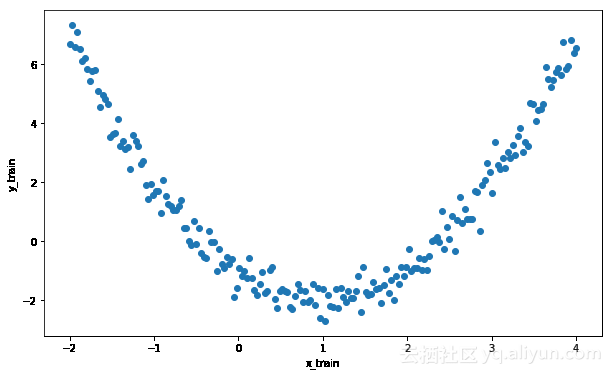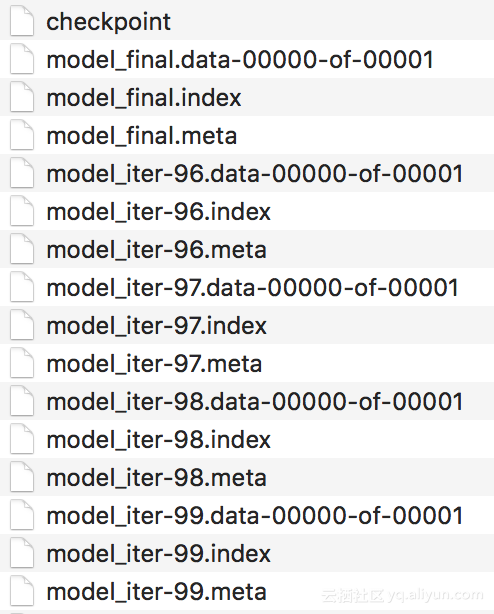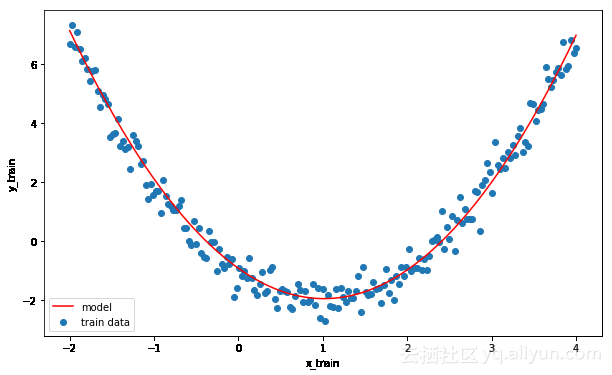# 深度学习小技巧（一）：如何保存和恢复TensorFlow训练的模型

+关注继续查看

1.首先我们将快速介绍TensorFlow模型

TensorFlow的主要功能是通过张量来传递其基本数据结构类似于NumPy中的多维数组，而图表则表示数据计算。它是一个符号库，这意味着定义图形和张量将仅创建一个模型，而获取张量的具体值和操作将在会话（session）中执行，会话（session）一种在图中执行建模操作的机制。会话关闭时，张量的任何具体值都会丢失，这也是运行会话后将模型保存到文件的另一个原因。

import tensorflow as tf
import numpy as np
import matplotlib.pyplot as plt
%matplotlib inline

y = (x - h) ^ 2 + v  

# Clear the current graph in each run, to avoid variable duplication
tf.reset_default_graph()
# Create placeholders for the x and y points
X = tf.placeholder("float")
Y = tf.placeholder("float")
# Initialize the two parameters that need to be learned
h_est = tf.Variable(0.0, name='hor_estimate')
v_est = tf.Variable(0.0, name='ver_estimate')
# y_est holds the estimated values on y-axis
y_est = tf.square(X - h_est) + v_est
# Define a cost function as the squared distance between Y and y_est
cost = (tf.pow(Y - y_est, 2))
# The training operation for minimizing the cost function. The
# learning rate is 0.001
trainop = tf.train.GradientDescentOptimizer(0.001).minimize(cost) 

# Use some values for the horizontal and vertical shift
h = 1
v = -2
# Generate training data with noise
x_train = np.linspace(-2,4,201)
noise = np.random.randn(*x_train.shape) * 0.4
y_train = (x_train - h) ** 2 + v + noise
# Visualize the data
plt.rcParams['figure.figsize'] = (10, 6)
plt.scatter(x_train, y_train)
plt.xlabel('x_train')
plt.ylabel('y_train')2.The Saver class

Saver类是TensorFlow库提供的类，它是保存图形结构和变量的首选方法。

2.1保存模型

# Create a Saver object
saver = tf.train.Saver()

init = tf.global_variables_initializer()

# Run a session. Go through 100 iterations to minimize the cost
def train_graph():
with tf.Session() as sess:
sess.run(init)
for i in range(100):
for (x, y) in zip(x_train, y_train):

# Feed actual data to the train operation
sess.run(trainop, feed_dict={X: x, Y: y})

# Create a checkpoint in every iteration
saver.save(sess, 'model_iter', global_step=i)

# Save the final model
saver.save(sess, 'model_final')
h_ = sess.run(h_est)
v_ = sess.run(v_est)
return h_, v_


result = train_graph()
print("h_est = %.2f, v_est = %.2f" % result)

$python tf_save.py h_est = 1.01, v_est = -1.96  Okay，参数是非常准确的。如果我们检查我们的文件系统，最后4次迭代中保存有文件以及最终的模型。 保存模型时，你会注意到需要4种类型的文件才能保存： “.meta”文件：包含图形结构。 “.data”文件：包含变量的值。 “.index”文件：标识检查点。 “checkpoint”文件：具有最近检查点列表的协议缓冲区。图1：检查点文件保存到磁盘 调用tf.train.Saver()方法，如上所示，将所有变量保存到一个文件。通过将它们作为参数，表情通过列表或dict传递来保存变量的子集，例如：tf.train.Saver({'hor_estimate': h_est}) Saver构造函数的一些其他有用的参数，也可以控制整个过程，它们是： 1.max_to_keep：最多保留的检查点数。 2.keep_checkpoint_every_n_hours：保存检查点的时间间隔。 如果你想要了解更多信息，请查看官方文档Saver类，它提供了其它有用的信息，你可以探索查看。 3.Restoring Models 恢复TensorFlow模型时要做的第一件事就是将图形结构从“.meta”文件加载到当前图形中。 tf.reset_default_graph() imported_meta = tf.train.import_meta_graph("model_final.meta")  也可以使用以下命令探索当前图形tf.get_default_graph()。接着第二步是加载变量的值。提醒：值仅存在于会话（session）中。 with tf.Session() as sess: imported_meta.restore(sess, tf.train.latest_checkpoint('./')) h_est2 = sess.run('hor_estimate:0') v_est2 = sess.run('ver_estimate:0') print("h_est: %.2f, v_est: %.2f" % (h_est2, v_est2)) $ python tf_restore.py
INFO:tensorflow:Restoring parameters from ./model_final
h_est: 1.01, v_est: -1.96  

plt.scatter(x_train, y_train, label='train data')
plt.plot(x_train, (x_train - h_est2) ** 2 + v_est2, color='red', label='model')
plt.xlabel('x_train')
plt.ylabel('y_train')
plt.legend()Saver这个类允许使用一个简单的方法来保存和恢复你的TensorFlow模型（图形和变量）到/从文件，并保留你工作中的多个检查点，这可能是有用的，它可以帮助你的模型在训练过程中进行微调。

4.SavedModel格式（Format）

4.1使用SavedModel Builder保存模型

tf.reset_default_graph()
# Re-initialize our two variables
h_est = tf.Variable(h_est2, name='hor_estimate2')
v_est = tf.Variable(v_est2, name='ver_estimate2')

# Create a builder
builder = tf.saved_model.builder.SavedModelBuilder('./SavedModel/')

# Add graph and variables to builder and save
with tf.Session() as sess:
sess.run(h_est.initializer)
sess.run(v_est.initializer)
[tf.saved_model.tag_constants.TRAINING],
signature_def_map=None,
assets_collection=None)
builder.save()  

$python tf_saved_model_builder.py INFO:tensorflow:No assets to save. INFO:tensorflow:No assets to write. INFO:tensorflow:SavedModel written to: b'./SavedModel/saved_model.pb'  运行此代码时，你会注意到我们的模型已保存到位于“./SavedModel/saved_model.pb”的文件中。 4.2使用SavedModel Loader程序恢复模型 模型恢复使用tf.saved_model.loader，并且可以恢复会话范围中保存的变量，符号。 在下面的例子中，我们将加载模型，并打印出我们的两个系数（h_estv_est）的数值。数值如预期的那样，我们的模型已经被成功地恢复了。 with tf.Session() as sess: tf.saved_model.loader.load(sess, [tf.saved_model.tag_constants.TRAINING], './SavedModel/') h_est = sess.run('hor_estimate2:0') v_est = sess.run('ver_estimate2:0') print("h_est: %.2f, v_est: %.2f" % (h_est, v_est)) $ python tf_saved_model_loader.py
INFO:tensorflow:Restoring parameters from b'./SavedModel/variables/variables'
h_est: 1.01, v_est: -1.96  

5.结论37 04378 0TensorFlow 学习指南 二、线性模型

1200 01038 01488 0Tensorflow加载预训练模型和保存模型
7097 01418 01620 01650 02065 0
【方向】

706

5